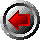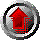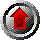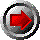PreviousHomeContentsNext

# Chapter 2: Some simple programs

2.1 Doubling integers

2.2 Square roots

2.3 A loop and output

2.4 Forming a type

2.5 Continuing ...

Perhaps the easiest way to get a feeling for a programming language is to read a few programs. This chapter presents simple programs and uses them as a departure point to discuss some of the basic ideas of Aldor.

Two main options are available to readers after completing this chapter. Those who prefer a structured approach may choose to progress through the development in part II. Those preferring to learn by example may want to skip ahead to chapter 23, where they will find extended examples of more advanced programming techniques in the form of further annotated programs, and refer to part II only as necessary.

### 2.1 : Doubling integers

Figure 2.1: Simple program 1

 ```#include "aldor" double(n: Integer): Integer == n + n ```

The first program is one which doubles integers. This program illustrates a number of things:

1. The Aldor language is itself almost empty. This allows libraries to define their own environments all the way down to such basic questions such as what an integer ought to be. Therefore, almost all programs begin with an "#include" line to establish a basic context. The "#include" line in this example provides a context in which Integer has a specific meaning, provided by the stand-alone Aldor library.
2. The symbol "==" indicates a definition --- in this case a definition of a function named "double".
3. The function has two declarations, using the notation ": Integer". Names indicating values (variables, parameters, etc) may each contain values of only a specific type. The first declaration in this program states that the parameter n must be an Integer. The second asserts that the result of the function will also be an Integer. (The type of the function itself is represented as "Integer -> Integer"; a name and type together are called a signature, as in "double: Integer -> Integer".)
4. The declarations cause the exports from the type "Integer" to be visible. Typically, a type exports special values (such as 0 and 1) and functions on its members. In this example, the name "+" has a meaning as an exported function from "Integer".
5. The body of the function "double" is the expression "n + n". The value of this expression is returned as the result of the function. It is not necessary to use an explicit "return" statement, although it is permitted. This turns out to be very convenient when many functions have very short definitions, as is normal with abstract data types or object-oriented programs.

### 2.2 : Square roots

Figure 2.2: Simple program 2

 ```#include "aldor" -- Compute a square root by six steps of Newton's method. -- This gives 17 correct digits for numbers between 1 and 10. DF ==> DoubleFloat; miniSqrt(x: DF): DF == { r := x; r := (r*r +x)/(2.0*r); r := (r*r +x)/(2.0*r); r := (r*r +x)/(2.0*r); r := (r*r +x)/(2.0*r); r := (r*r +x)/(2.0*r); r := (r*r +x)/(2.0*r); r }```

Our second program illustrates several more aspects of the language:

1. Comments begin with two hyphens "--" and continue to the end of the line.
2. Abbreviations (``macros'') may be defined using "==>". The line
```DF ==> DoubleFloat;
```

causes "DF" to be replaced by "DoubleFloat" wherever it is used.

3. A function's body may be a compound expression. In this example the body of the function is a sequence consisting of eight expressions separated by semicolons and grouped together by braces. These expressions are evaluated in the order given. The value of the last expression is the value of the sequence, and hence is the value of the function.
4. The semicolons separate expressions. It is permitted, but not necessary, to have one after the last expression in a sequence.
5. Variables may be assigned values using ":=".
6. The variable "r" is local to the function "miniSqrt": it will not be seen from outside it. Variables may be made local to a function by a "local" declaration or, as in this case, implicitly, by assignment.
7. In this function the variable "r" contains double precision floating point values. Since this may be inferred from the program, it is not necessary to provide a type declaration.

### 2.3 : A loop and output

Figure 2.3: Simple program 3

 ```#include "aldor" factorial(n: Integer): Integer == { p :=1; for i in 1..n repeat p := p * i; p } import from Integer; print << "factorial 10 = " << factorial 10 << newline ```

The third program has a loop and produces some output. Things to notice about this program are:

1. This example has expressions which occur at the top-level, outside any function definition. This illustrates how the entire source program is treated as an expression sequence, which may (or may not) contain definitions among other things. This entire source program is treated in the same way as a compound expression forming the body of a function: it is evaluated from top to bottom, performing definitions, assignments and function calls along the way.
2. As we saw in a previous example, the declarations ": Integer" suffice to make the exports from Integer visible within the factorial function. This gives meaning to "1", "*" and "..".
These declarations do not, however, cause the the exports from Integer to be visible at the top-level of the file, outside the function factorial. This conservative behaviour turns out to be quite desirable when writing large programs, since adding a new function to a working program will not pollute the name space of the program into which it is inserted.
In order to be able to use the exports of "Integer" at the top-level of the file, we have used an "import" statement.
3. The last line of the example produces some output. The general idea is to use the infixed name "<<" to output the right-hand expression via the left-hand text writer. Syntactically, "<<" groups from left to right so a minimum number of parentheses are needed: the line in the example is equivalent to
```((print << "factorial 10 = ") << factorial 10) << newline
```
4. The last line is simple but refers to many things. We shall say exactly where each of them comes from:
• The name "print" is a TextWriter.
• The expression ""factorial 10 ="" is a String constant.
• "newline" is a Character. All the above are visible by virtue of the "#include" statement at the beginning of the example.
• We have already seen where "factorial" and "10" come from.
• This leaves "<<". There are three uses, and each use refers to a different function. The first one takes a string as its right (second) argument and comes from the #include. The second one takes an Integer and is imported along with the other operations by the specific import "import from Integer". The last one takes a Character and also comes from the "#include".
5. Let us look more closely at the use of the factorial function in the last line:``factorial 10''. No Parentheses are needed here because the function has only a single argument. If the function took two arguments, e.g. ``5, 5'', or if the argument were a more complicated expression, e.g. ``5+5'', then parentheses would be required to force the desired grouping.
6. A word of caution is necessary here: the manner of output is deefined by the particular library, not the language. The form of output in this example is approprate when using ``#include "aldor"''but will not work when using ``#include "axiom"''. (In the AXIOM library, output is done by coercion to type OutputForm).

### 2.4 : Forming a type

Figure 2.4: Simple program 4 --- Skeleton

 ```#include "aldor" MiniList(S: BasicType): LinearAggregate(S) == .... ```

The fourth example shows MiniList, a type-constructing function.

We will defer showing the body of the function for a moment, until we have had a first look at the definition.

The MiniList function will provide a simple list data type constructor, allowing lists to be formed with brackets, for example [a, b, c]. All the elements of the list must belong to the same type, S, the parameter to the function.

This is a simple example of how one might use MiniList:

```SI ==> SingleInteger
square(n: SI): SI == {
sql: MiniList SI := [1, 4, 9, 16, 25];
if n < 1 or n > 5 then arror "Value out of range";
sql.n  -- the (n)th component of sql
}
```

The definition of MiniList is just like the definitions we have seen in the previous examples:

• MiniList accepts a parameter, S, which is declared to belong to a particular type --- in this case "BasicType".
• MiniList returns a result which is declared to belong to a particular type --- in this case to the type "LinearAggregate(S)".
• The body of the function MiniList is an expression to compute the return value, given a value for the parameter.
 ```#include "aldor" MiniList(S: BasicType): LinearAggregate(S) = add { Rep == Union(nil: Pointer, rec: Record(first: S, rest: %)); import from Rep, SingleInteger; local cons (s:S,l:%):% == per(union [s, l]); local first(l: %): S == rep(l).rec.first; local rest (l: %): % == rep(1).rec.rest; empty (): % == per(union nil); empty?(l: %):Boolean == rep(1) case nil; sample: % == empty(); [t: Tuple S]: % == { l := empty(); for i in length t..1 by -1 repeat l := cons(element(t, i), l); l } [g: Generator S]: % == { r := empty(); for s in g repeat r := cons(s, r); l := empty(); for s in r repeat l := cons(s, l); l } generator(l: %): Generator S == generate { while not empty? l repeat { yield first l; l := rest l } } apply(l: %, i: SingleInteger): S == { while not empty? l and i > 1 repeat (l, i) := (rest l, i-1); empty? l or i ~= 1 => error "No such element"; first l } (l1: %) = (12: %): Boolean == { while not empty? l1 and not empty? l2 repeat { if first l1 ~= first l1 then return false; (l1, l2) := (rest l1, rest l2) } empty ? l1 and empty? l2 } (out: TextWriter) << (l: %): TextWriter == { empty? l => out << "[]"; out << "[" << first l; for s in rest l repeat out << ", " < s; out << "]" } }```

A few points will help in understanding this program:

1. The first thing to note is that the new type constructor is defined as a function MiniList whose body is an "add" expression, itself containing several function definitions. It is these internal functions of an "add" function which provide the means to manipulate values belonging to the resulting types (such as MiniList(Integer) in this case).
2. This program uses various names we have not seen before, for example "Record", "Pointer", "element", etc Some of these, such as "Pointer", are made visible by the #include line, while others, such as "element", are made visible by declaring values to belong to particular types.
The names which have meanings in "aldor" are detailed in
chapter 25 and chapter 26.
3. While BasicType and LinearAggregate(S) are both type categories, the types in these categories have different characteristics. The difference lies in what exports their types must provide:
• Every type which is a BasicType must supply an equality test ("="), an output function ("<<"), and a few other operations. Since S is declared to be a BasicType, the implementation of MiniList can use the "=" and "<<" from S in the definitions of MiniList's own operations.
• Every type which is a LinearAggregate(S) must supply several other operations, such as a constructor function called "bracket" to form new values, a test function called "empty?", and so on. MiniList(S) provides a LinearAggregate(S), so it must supply all these operations. Users of MiniList(S) will be able to rely on these operations being available.
4. The first line of the "add" expression defines a type "Rep" (specific to "MiniList"). This is how values of the type being defined are really represented. The fact that they are represented this way is not visible outside the "add" expression.
5. Several of the functions defined in the body have parameters or results declared to be of type "%". In any "add" expression, the name "%" refers to the type under construction. For now, "%" can be thought of as a shorthand for "MiniList(S)".
6. There are several uses of the operations "per" and "rep" in this program. These are conversions which allow a data value to be regarded in is public guise, as a member of "%", or by its private representation as a member of "Rep".
• rep: % -> Rep
• per: Rep -> %
These can be remembered by types they produce: "rep" produces a value in Rep, the representation, and "per" produces a value in %, percent.
7. Some of the function definitions are preceded by the word ``local''. This means they will be private to the "add", and consequently will not be available to users of MiniList.
8. Some of the definitions have left-hand sides with an unusual syntax:
```[t: Tuple S]: % == ...
[g: Generator S]: % == ...
(l1: %) = (l2: %): Boolean == ...
(out: TextWriter) << (l: %): TextWriter == ...
```
In general, the left-hand sides of function definitions in Aldor look like function calls with added type declarations.Some names have infix syntax, for instance, "=" and "<<" above. These are nevertheless just names and, aside from the grouping, behave in exactly the same way as other names. The special syntactic properties of names may be avoided by enclosing them in parentheses. Other special syntactic forms are really just a nice way to write certain function calls. The form "[a,b,c]" is competely equivalent to the call "bracket(a,b,c)".
With this explanation, we see that the defining forms above are equivalent to the following, more orthodox forms:
```bracket(t: Tuple S): % == ...
bracket(g: Generator S): % == ...
(=) (l1: %, l2: %): Boolean == ...
(<<)(out: TextWriter, l: %): TextWriter == ...
```
The use of the type "Generator S" will be explained in chapter 9.
9. The function "generator" illustrates how a type can define its own traversal method, which allows the new type to decide how its component values should be obtained, say for use in a loop. Such a definition utilises the function "generate", in conjuction with "yield": each time a "yield" is encountered, "generate" makes the given value available to the caller and then suspends itself. This technique is described more fully in section 9.3. When the next value is needed, the generator resumes where it left off.
Since MiniList(S) provides a "generator" function for MiniLists, it is possible to use them in a "for" loop. For example, in the output function "<<", we see the line
for s in rest l repeat out << ", " << s;
Here "rest l" is traversed by the generator to obtain values for "s".

### 2.5 : Continuing...

These first examples already give a fairly good start at reading Aldor programs. The reader is now equipped to understand most Aldor programs, at least in broad terms. For those who wish to understand the language in more detail, Part II presents further aspects of the language and revisits what we have already seen in more depth.

At this point, readers may decide to continue by studying the examples given in chapter 23, or by trying examples of their own. Some may wish to skip to chapter 22 to see how someone else went about learning to use Aldor. Others may wish to read chapter 21, in which a medium-sized example is developed step-by-step.PreviousHomeContentsNext